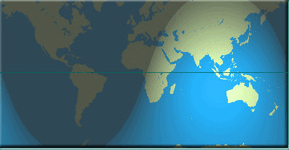# Linear references and 3D time again

This post is related to previous ones about base + 3D duration (3D time), such as here and here.

Time is measured in terms of the annual calendar and the daily clock. Clocks are based on the apparent motion of the Sun and other celestial bodies across the sky, divided into 24 hours. The calendar is based on the annual cycle of the Sun’s declination (the angular distance north or south of the celestial equator), divided into 12 months and 365 (or 366) days. Thus time is measured in terms of the Earth’s orbital and rotational periods.

Length may be measured similarly. The original definition of the metre (meter) in 1793 was one ten-millionth of the distance from the equator to the North Pole. What is required for linear references is a motion whose travel length may be accumulated indefinitely. What corresponds to the clock could be the motion of the night shadow across the face of the Earth at the equator:So the evening and the morning were the [Nth] day. Genesis 1

The circumference of the Earth is 40,075,000 m. This is the distance moved by the edge of the day-night boundary in 24 hours. Then one hour is equivalent to 1,669,792 m and one second is equivalent to 464 m. The shadow motion may be accumulated indefinitely to make reference length, similar to reference time.

Whichever dimension is limited to 1D (radial measure) is the measure of a motion from the perspective of the body in motion, whether this is measured by the length or duration. Since the 1D variable is the independent variable, this is selected as a standard motion available (in principle) to everyone. So the 1D variable becomes a universal radial distance (stance) or radial time against which to measure motions in 3D of duration or length, respectively.